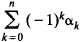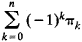# Euler Characteristic

(redirected from Euler-Poincare characteristic)
The following article is from The Great Soviet Encyclopedia (1979). It might be outdated or ideologically biased.

## Euler Characteristic

The Euler characteristic of a polyhedron is the number α0 — α1 + α2, where α0 is the number of vertices, α1 is the number of edges, and α2 is the number of faces. According to Euler’s theorem, if the polyhedron is convex or is homeomorphic to a convex polyhedron, then its Euler characteristic is 2. This fact was known to R. Descartes; L. Euler published a proof of the theorem in 1758.

The Euler characteristic of an arbitrary simplicial complex is the numberwhere n is the dimension of the complex, α0 is the number of vertices, and α1 is the number of edges. In general, k is the number of k-dimensional simplexes belonging to the complex. According to the Euler-Poincaré formula, the Euler characteristic is equal towhere πk is the k-dimensional Betti number of the complex. The topological invariance of the Euler characteristic follows from this fact.

In view of the topological invariance of the Euler characteristic, we speak of the Euler characteristic of a surface and of a polytope, by which we mean the Euler characteristic of any triangulation of the surface or polytope.

### REFERENCES

Aleksandrov, P. S. Kombinatornaia topologiia. Moscow-Leningrad, 1947.
Pontriagin, L. S. Osnovy kombinatornoi topologii, 2nd ed. Moscow, 1976.
References in periodicals archive ?
Different types of microdomains have different values of the local Euler-Poincare characteristic. That demonstrates this measure can discriminate well between different morphologies (Fig.
This set of MFs includes volume V, surface S and Euler-Poincare characteristic (or connectivity number x), obtained with Gwyddion 2.28 software, and can be expressed as (46):
An integral-geometric approach for the Euler-Poincare characteristic of spatial images.
Therefore, when dimM = 2, (M, g) admits an orthogonally conformal vector field if, and only if, either M is noncompact or M is compact and its Euler-Poincare characteristic vanishes.
[S] Ch VII) proved the existence of a moduli space U(C, n, d, [[Lambda].sub.i]) parametrising [[Lambda].sub.i]-stable sheaves on C of given ranks [r.sub.i] and given Euler-Poincare characteristic. Its natural compactification is the set of equivalence classes of semistable vector bundles on C.
In all what follows we fix a rank n (the same for all components), an Euler-Poincare characteristic [Chi] = d + n(1 - g) and weights [[Lambda].sub.i].
Local contributions to the Euler-Poincare characteristic of a set.
An integralgeometric approach for the Euler-Poincare characteristic of spatial images.
Minkowski functionals encompass standard geometric parameters such as volume, area, length and the Euler-Poincare characteristic. Software tools for computing approximations of Minkowski functionals on binary 2D or 3D images are now available based on mathematical methods due to Serra, Lang and Ohser.
The Euler-Poincare characteristic is a standard connectivity parameter.
Some of the intrinsic volumes have a nice geometric interpretation: [V.sub.d](K) is the usual volume of K, d[V.sub.d[??]1](K) is the surface area of K and [V.sub.0](K) is the Euler-Poincare characteristic of K.
Sujatha, Euler-Poincare characteristics of abelian varieties, C.

Site: Follow: Share:
Open / Close